# Exponential convertion - the useful formulas

The exponential converter / logarithmic converter is a very useful circuit, used for converting a linear CV to a logarithmic current in analog synthesizer VCOs and VCAs etc. They are usually build by exploiting a fundamental property of transistors where the transistor output current is a natural exponential function of the voltage between the transistor base and emitter. Single transistor exponential converters however, are terribly temperature sensitive, so a dual transistor scheme that compensates for many of the temperature effects is often used.

This article describes four different variations of this circuit. I have seen several articles saying that you can change what transistor to connect the reference current to, and what base to use for controlling the converter. This text explains how. I will not go into details about how they compensate for temperature variations, but I will give you the necessary formulas and explain how I arrived at them.

Check out my three articles on the VCO for explanations of how temperature compensation actually works.

## The four configurations are:

• A: Decreasingly positive exponential (starting high positive and decreasing to close to 0)
• B: Increasingly positive exponential (starting close to 0 and increasing to high positive)
• C: Increasingly negative exponential (starting close to 0 and increasing to high negative)
• D: Decreasingly negative exponential (starting high negative and decreasing to close to 0)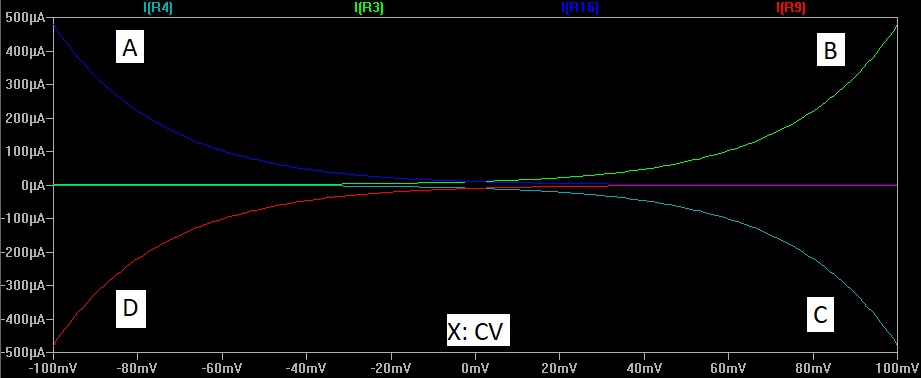The four plots are derived from the same $-100mV$ to $+100mV$ control voltage (CV).

## The test circuit looks like this: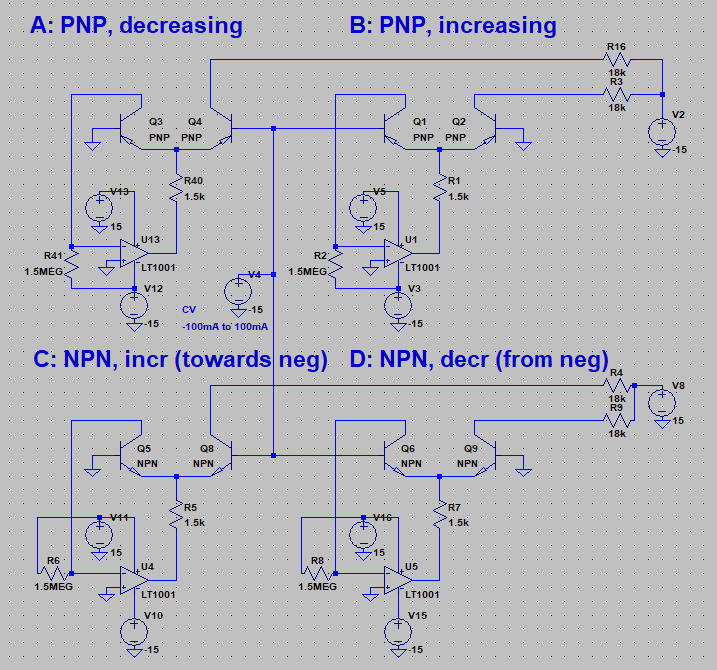NB: -100mA to 100mA should be -100mV to 100mV, this is an error in the image.

There are only tiny variations in the circuits:

• For each exponential converter, a reference current is connected to the left transistor's collector.
• A and C have the CV connected to the right transistor's base (the other is grounded)
• B and D have the CV connected to the left transistor's base (the other is grounded)
• A and B are PNP transistors. For these, the reference current must be negative, i.e. flow out of the transistor's collector.
• A and B are NPN transistors. For these, the reference current must be positive, i.e. flow into the transistor's collector.

## These are the formulas for the four exponential converters:

• A: $I_{out} = I_{ref} \cdot e^{-\frac{V_{cv}}{V_T}}$
• B: $I_{out} = I_{ref} \cdot e^{\frac{V_{cv}}{V_T}}$
• C: $I_{out} = I_{ref} \cdot e^{\frac{V_{cv}}{V_T}}$
• D: $I_{out} = I_{ref} \cdot e^{-\frac{V_{cv}}{V_T}}$

$V_T = \frac{k \cdot T}{q}$

$k = 1.38 \cdot 10^{-23}$ Joules/Kelvin
$q = 1.6 \cdot 10^{-19}$ Coloumbs
$T =$ absolute temperature in degrees Kelvinq (= degrees celcius + 273.16)

$V_T$ is $25.7mV$ at 25°C - see other documents on this site for temperature compensation

It is also important to note that

• $I_{ref}$ and $I_{out}$ flows INTO the collectors for A and B
• $I_{ref}$ and $I_{out}$ flows OUT OF the collectors for C and D

$I_{ref}$ is generated by the $1.5MOhm$ resistors connected to + or - $15V$ in the test circuit. Let's call the resistor $R_{ref}$ and the voltage $V_{ref}$.

The reference current $I_{ref}$ is then:

$I_{ref} = \frac{V_{ref}}{R_{ref}}$

or $\frac{1.5MOhm}{15V} = 10uA$ for C and D, $\frac{1.5MOhm}{-15V} = -10uA$ for A and B, meaning the current flows out of the opamp's negative input and towards -15V for A and B.

## Things to be aware of

• The output of the converter is a current, not a voltage
• The reference current sets the output current when $V_{cv}$ = 0
• The output current is doubled/halved by increasing/decreasing $V_{cv}$ by approx $17.5mV$. The exact value is temperature dependent. Doubling or halving the current is important, as a frequency change of one octave is equal to doubling or halving of the frequency.
• Design the pre-$V_{cv}$-input circuitry to produce a $17.5mV$ response that matches your wanted CV response - e.g. if your input CV is 0 to 5V and you want a $1oct/V$ response, you need to make a circuit with $V_{out} = V_{in} \cdot 0.0175$.
• Add or subtract a voltage from the input CV to trim the starting points of octaves (tune a note)
• The voltage at the collector of the right transistor, $V_{c_2}$, cannot be determined by looking at the exponential converter alone - it depends on whatever you put after the converter. However, for the transistor to conduct, $V_c$ must be at least $0.25V$ (approx) larger than $V_e$ for the NPN configuration, and $V_c$ must be at least $0.25V$ (approx) less than $V_e$ for the PNP configuration. This limits the maximum $I_{out}$, see example at the end.

# Facts and background information

• Two transistors with connected emitters have the same $V_e$.
• The collector current $I_c$ is directly related to $V_{be}$
[see how]
The collector current $I_c$ is directly related to voltage difference between the base and the emitter, $V_{be}$, through the Ebers-Moll equations. If one changes, the other changes. The only way $V_{be}$ can change is if $I_c$ also changes (and thus, changing $V_{be}$ changes $I_c$). TODO:and not to be found using the hef or something.
• $I_{ref}$ is constant (in our circuits)
[see how]
If we just connected s resistor to the left collector and hoped to get s constant $I_{ref}$, we would be disappointed. $V_{c_1}$ will vary and thus $I_{ref}$ varies too. A constant $I_{ref}$ is generated by connecting a resistor $R_{ref}$ between a known, constant voltage and the collector of one of the transistors. The transistor is then placed within the feedback loop of an opamp, with the collector connected to the negative input and the emitter connected (through a resistor) to the opamp output. As the collector is now at virual ground, the current flowing through $R_{ref}$ is $\frac{V_{ref}}{R_{ref}}$. This same current flows through the collector, as the opamp tries to make the sum of currents into its inputs zero.

Now let's figure out the exact formulas for the relationship between the two transistors' $V_b$ and $I_c$:

### Deducing the NPN equationCurrents and voltages in the NPN version. In the following text $I_{ref} = I_{c_1}$ and $I_{out} = I_{c_2}$, $V_{be_x} = V_{b_x} - V_e$.

The Ebers-Moll equation for an NPN transistor can be approximated as

$I_c = I_s \cdot e^{\frac{V_{be}}{V_T}}$

Rearranging for $V_{be}$ gives us

$V_{be} = V_T \cdot ln(\frac{I_c}{I_s})$

Here's a little trick. Since $V_e$ is the same for both transistors, the difference in $V_{be}$ between the two transistors can be written as

$\Delta V_{be} = V_{be_2} - V_{be_1}$
$\Delta V_{be} = (V_{b_2} - V_e) - (V_{b_1} - V_e)$
$\Delta V_{be} = V_{b_2} - V_{b_1}$

The order of the bases in the delta is unimportant, as long as we use the same ordering for both voltages and currents we will end up at the same result.

Also

$\Delta V_{be} = V_T \cdot ln(\frac{I_{c_2}}{I_s}) - V_T \cdot ln(\frac{I_{c_2}}{I_s})$
$\Delta V_{be} = V_T \cdot (ln(\frac{I_{c_2}}{I_s}) -ln(\frac{I_{c_2}}{I_s})$
$\Delta V_{be} = V_T \cdot ln(\frac{I_{c_2}}{I_{c_1}})$

So we get

$V_{b_2} - V_{b_1} = V_T \cdot ln(\frac{I_{c_2}}{I_{c_1}})$
$ln(\frac{I_{c_2}}{I_{c_1}}) = \frac{V_{b_2} - V_{b_1}}{V_T}$
$\frac{I_{c_2}}{I_{c_1}} = e^{\frac{V_{b_2} - V_{b_1}}{V_T}}$

For NPN:

$I_{c_2} = I_{c_1} \cdot e^{\frac{V_{b_2} - V_{b_1}}{V_T}}$

### Deducing the PNP equationCurrents and voltages in the PNP version. In the following text $I_{ref} = I_{c_1}$ and $I_{out} = I_{c_2}$, $V_{be_x} = V_{b_x} - V_e$

The Ebers-Moll equation for a PNP transistor on the other hand can be approximated as

$I_c = I_s \cdot e^{\frac{V_{eb}}{V_T}}$

[show where the PNP equation came from]

### Where did the PNP equation come from?

The NPN transistor's forward characteristics are, according to Microelectronic Circuit Design by Travis Blalock, Richard Jaeger - http://www.jaegerblalock.com/

$I_c = I_s \cdot (e^{\frac{V_{be}}{V_T}} - 1) = \alpha_F I_{ES} \cdot (e^{\frac{V_{be}}{V_T}} - 1)$ (5.21)

and thus

$I_{ES} = \frac{I_s}{\alpha_F}$

which is also stated in 5.20

The forward characteristics for the PNP are not clearly stated, but the Ebers-Moll Models for both tne NPN and the PNP are. For NPN it is:

$I_c = \alpha_F I_{ES} \cdot (e^{\frac{V_{be}}{V_T}} - 1) - I_{cs} \cdot (e^{\frac{V_{bc}}{V_T}} - 1)$

Here, we recognize the first part as the forward characteristic and the second as the reverse characteristic (5.22).

For PNP it is

$I_c = \alpha_F I_{ES} \cdot (e^{\frac{V_{eb}}{V_T}} - 1) - I_{cs} \cdot (e^{\frac{V_{cb}}{V_T}} - 1)$

We know that the first part is the forward characteristic, so

$I_c = \alpha_F I_{ES} \cdot (e^{\frac{V_{eb}}{V_T}} - 1)$, and $I_{ES} = \frac{I_s}{\alpha_F}$

which means that the forward characteristic for the PNP is

$I_c = (e^{\frac{V_{eb}}{V_T}} - 1)$

Note that the only difference is the way we calculate the difference between $V_b$ and $V_e$ - a tiny but crucial difference.

Rearranging for $V_{be}$ gives us

$V_{eb} = V_T \cdot ln(\frac{I_c}{I_s})$

Then the same trick: Since $V_e$ is the same for both transistors, the difference in $V_{be}$ between the two transistors can be written as

$\Delta V_{eb} = V_{eb_2} - V_{eb_1}$
$\Delta V_{eb} = (V_e - V_{b_2}) - (V_e - V_{b_1})$
$\Delta V_{eb} = -V_{b_2} + V_{b_1}$
$\Delta V_{eb} = V_{b_1} + V_{b_2}$

Similarly,

$\Delta V_{eb} = V_T \cdot ln(\frac{I_{c_2}}{I_s}) - V_T \cdot ln(\frac{I_{c_2}}{I_s})$
$\Delta V_{eb} = V_T(ln(\frac{I_{c_2}}{I_s}) -ln(\frac{I_{c_2}}{I_s})$
$\Delta V_{eb} = V_T \cdot ln(\frac{I_{c_2}}{I_{c_1}})$

So we get

$V_{b_1} - V_{b_2} = V_T \cdot ln(\frac{I_{c_2}}{I_{c_1}})$
$ln(\frac{I_{c_2}}{I_{c_1}}) = \frac{V_{b_1} - V_{b_2}}{V_T}$
$\frac{I_{c_2}}{I_{c_1}} = e^{\frac{V_{b_1} - V_{b_2}}{V_T}}$

For PNP:

$I_{c_2} = I_{c_1} \cdot e^{\frac{V_{b_1} - V_{b_2}}{V_T}}$

We now have the formulas for both PNP and NPN transistors. They say nothing about which one of the collectors are to be considered the reference current, nor which one, if any of, the bases are grounded or which one is the control input. If we want to find $I_{c_1}$ based on $I_{c_2}$ instead, we just negate the exponent:

$a = b \cdot e^{x} => b = \frac{a}{e^{x}} = a \cdot \frac{1}{e^{x}} = a \cdot e^{-x}$

## What is going on in the circuits?

Before the final transformation into the formulas above, let's stop to think about what is actually going on in the circuits.

The base of one of the transistors has been grounded in each circuit. Why?

### If we ground the base of the transistor that is inside the feedback loop (A, C):

• We now have a transistor with a fixed $V_{b_1}$ and a fixed $I_{c_1}$ ($I_{ref}$). As mentioned, a fixed $V_c$ means a fixed $V_{be}$, and since $V_b$ is also fixed, $V_e$ will be constant. It cannot go anywhere.
• Therefore, as we change $V_{b_2}$ (the CV), the difference between the base of the right transistor and the shared emitter, $V_{be_2}$, will change, and $I_{c_2}$ ($I_{out}$) changes.

### For A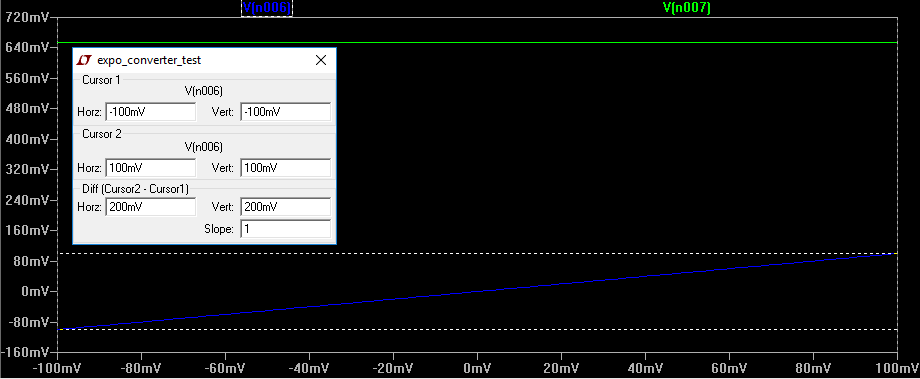$V_e$ (green) vs $V_{b_2}$ ($=V_{cv}$, blue). For a PNP transistor, the emitter voltage is higher than the base voltage when the transistor is on. The emitter voltage stays constant so the difference between the emitter and the base, $V_{be}$, decreases when $V_{cv}$ increases.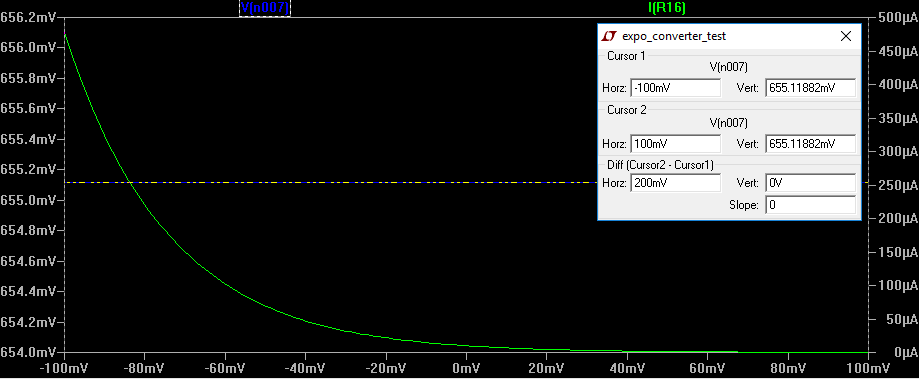Green line is $I_{out}$, dotted line is $V_e$. As $V_{cv}$ increases and thus $V_{be}$ decreases, the output current $I_{out}$ decreases.

### For C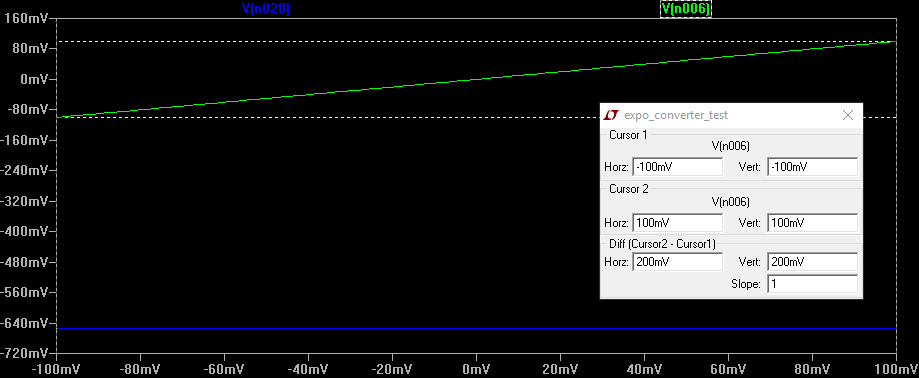$V_e$ (blue) vs $V_{b_2}$ ($=V_{cv}$, green). For an NPN transistor, the emitter voltage is lower than the base voltage when the transistor is on. The emitter voltage stays constant so the difference between the emitter and the base, $V_{be}$, increases when $V_{cv}$ increases.Green line is $I_{out}$, dotted line is $V_e$. As $V_{cv}$ increases and thus $V_{be}$ increases, the output current $I_{out}$ increases. As the reference current is flowing into the transistor, the output is also a negative current.

### If we ground the base of the transistor connected to the output (B, D):

• The transistor inside the feedback loop still has a fixed $I_{c_1}$, which means that $V_{be_1}$ is also fixed. But now the CV is connected to the base, i.e. $V_{b_1}$ is variable. Thus, to keep $I_{c_1}$ constant, $V_e$ must also change.
• The other transistor however, has its base grounded. $V_{b_2}$ is constant. Now, as $V_{b_1}$ changes, $V_e$ changes. This in turn changes difference between the right base and the emmiter, $V_{be_2}$, and as $V_{be_2}$ changes, $I_{c_2}$ ($I_{out}$) changes.

### For B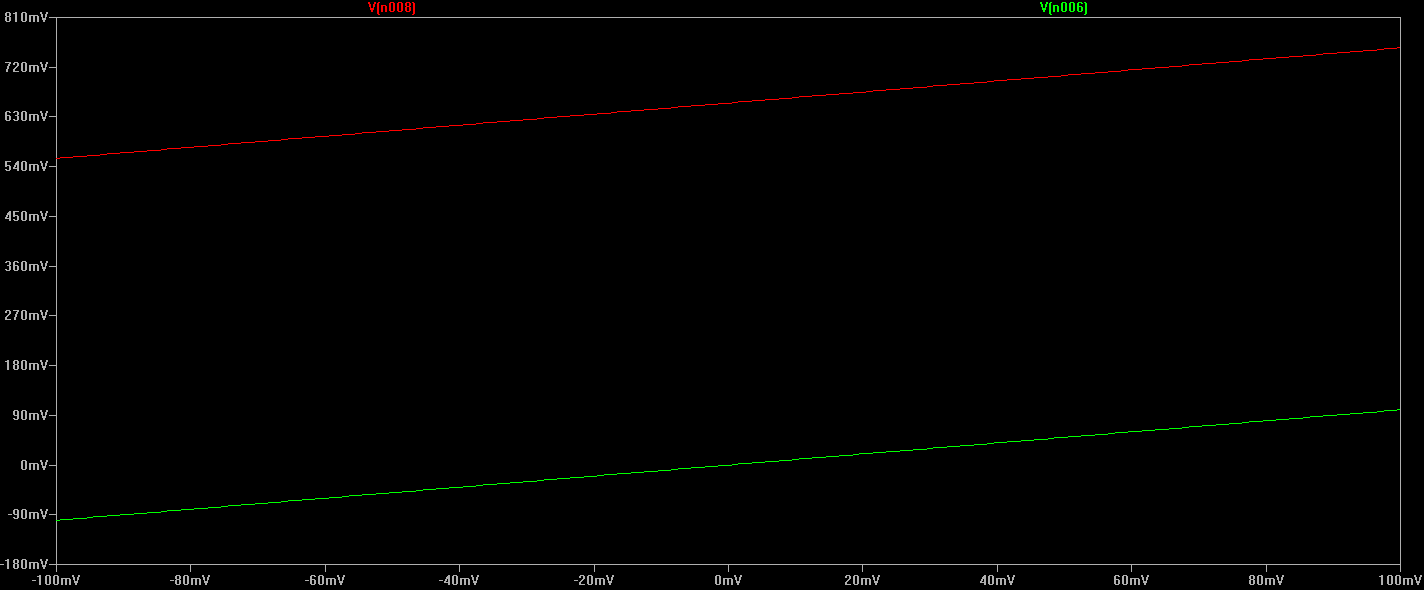$V_e$ (red) vs $V_{b_1}$ ($=V_{cv}$, green). For a PNP transistor, the emitter voltage is higher than the base voltage when the transistor is on. The difference between the left base voltage $V_{b_1}$ and the emitter voltage $V_e$ stays constant, but $V_{b_1}$ (=$V_{cv}$) changes, so $V_e$ changes with the same amount. The right base, $V_{b_2}$ is tied to 0, so as the emitter voltage increases, the difference between the emitter and the right base, $V_{be_2}$, increases.Blue line is $I_{out}$, green line is $V_e$. As $V_{cv}$ increases and thus $V_{be_2}$ increases, the output current $I_{out}$ increases.

### For D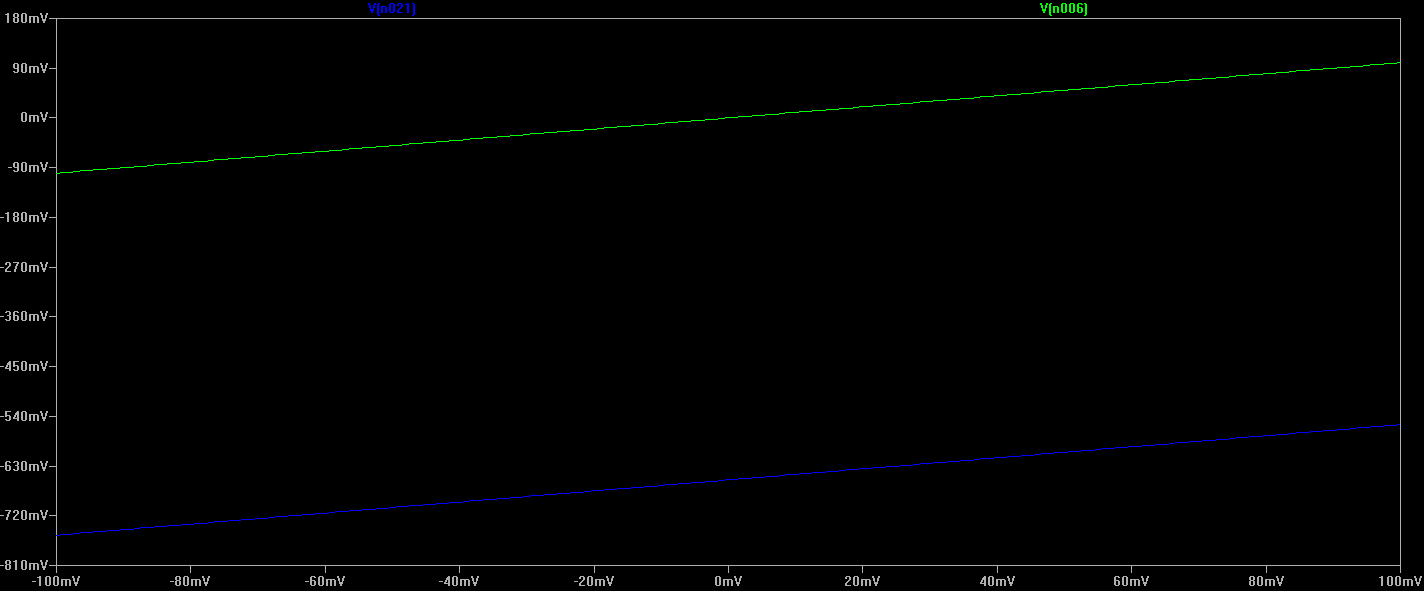For D: $V_e$ (green) vs $V_{b_1}$ ($=V_{cv}$, blue). For an NPN transistor, the emitter voltage is lower than the base voltage when the transistor is on. The difference between the left base voltage $V_{b_1}$ and the emitter voltage $V_e$ stays constant, but $V_{b_1}$ (=$V_{cv}$) changes, so $V_e$ changes with the same amount. The right base, $V_{b_2}$ is tied to 0, so as the emitter voltage increases, the difference between the emitter and the base, $V_{be_2}$, decreases.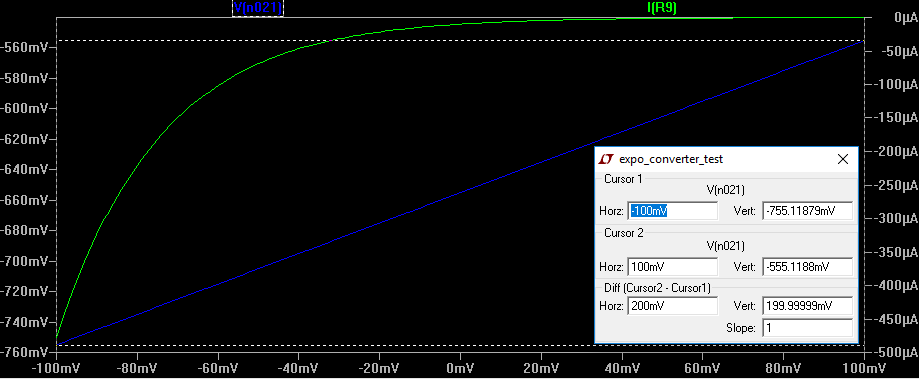Green line is $I_{out}$, blue line is $V_e$. As $V_{cv}$ increases and thus $V_{be_2}$ decreases, the output current $I_{out}$ decreases. As the reference current is flowing into the transistor, the output is also a negative current.

## The final transformation

Let's deduce the exact formulas for each of our circuits.

### For A

A is a PNP configuration, so we'll use

$I_{c_2} = I_{c_1} \cdot e^{\frac{V_{b_1} - V_{b_2}}{V_T}}$

If we set

$I_{c_2} = I_{out}$
$I_{c_1} = I_{ref}$
$V_{b_1} = 0$ (grounded)
$V_{b_2}$ is our control, $V_{cv}$

we're left with

A: $I_{out} = I_{ref} \cdot e^{-\frac{V_{cv}}{V_T}}$

### For B

B is also a PNP configuration, so we'll use

$I_{c_2} = I_{c_1} \cdot e^{\frac{V_{b_1} - V_{b_2}}{V_T}}$

If we set

$I_{c_2} = I_{out}$
$I_{c_1} = I_{ref}$
$V_{b_1}$ is our control, $V_{cv}$
$V_{b_2} = 0$ (grounded)

we're left with

B: $I_{out} = I_{ref} \cdot e^{\frac{V_{cv}}{V_T}}$

### For C

C is an NPN configuration, so we'll use

$I_{c_2} = I_{c_1} \cdot e^{\frac{V_{b_2} - V_{b_1}}{V_T}}$

If we set

$I_{c_2} = I_{out}$
$I_{c_1} = I_{ref}$
$V_{b_1} = 0$ (grounded)
$V_{b_2}$ is our control, $V_{cv}$

we're left with

C: $I_{out} = I_{ref} \cdot e^{\frac{V_{cv}}{V_T}}$

### For D

D is also an NPN configuration, so we'll use

$I_{c_2} = I_{c_1} \cdot e^{\frac{V_{b_2} - V_{b_1}}{V_T}}$

If we set

$I_{c_2} = I_{out}$
$I_{c_1} = I_{ref}$
$V_{b_1}$ is our control $V_{cv}$
$V_{b_2} = 0$ (grounded)

we're left with

D: $I_{out} = I_{ref} \cdot e^{-\frac{V_{cv}}{V_T}}$

## Max $I_{out}$

As mentioned, $V_{c_2}$ is determined by $I_{out}$ and the circuit after the collector. Let's look at an example:

Here, $I_{out}$ is fed through an 18k resistor. Following Ohms law, the voltage across the resistor has to be $U = R \cdot I = 18kOhm \cdot I_{out}$. As the bottom of the resistor is tied to $-15V$, the side connected to the collector must have a voltage $V_{c_2} = -15V + 18kOhm \cdot I_{out}$.

As also mentioned, $V_c$ for a PNP transistor must be at least $0.25V$ less than $V_e$. It is thus the value of $V_e$ that limits the maximum $I_{out}$:

$I_{out_{max}} = \frac{(V_e - 0.25) + 15V}{18kOhm}$, and $V_e$ is a result of $I_{ref}$.This shows what happens as the output current for B increases. The red line is $V_{b_1}$, the green is $V_e$. They are both increasing though it is hard to see in this image. As a result of this, $I_{out}$, the light blue line, also increases. This means that to adhere to Ohms law, the voltage across the 18k resistor in the test circuit increases too, which in turn means that $V_{c_2}$ increases - this is the dark blue line . At around $V_{b_1}=115mV$ the difference between the collector voltage and the emitter voltage is no longer > 0.25V and the transistor won't conduct any larger current. Thus the max $I_{out}$ has been reached.

## $R_e$

$R_e$ limits the current that flows into the constant current-generator op amp. To keep the current constant, it has to sink (or source) exactly $I_{ref} + I_{out}$. It does this by moving its output voltage up and down. The voltage across $R_e$ follows Ohms law, $V_{R_e} = R_e \cdot I_e = R_e \cdot (I_{ref} + I_{out})$. When $V_{R_e}$ gets too large, the opamp cannot pull its output further up or down, and $I_{out}$ cannot get any higher. The smaller the value for $R_e$, the less voltage across it.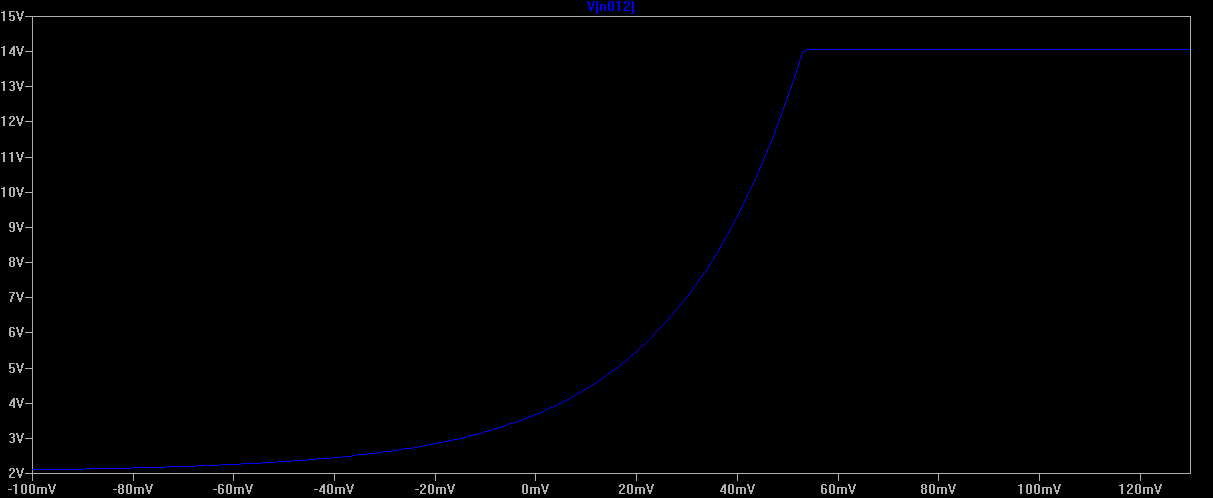Here we have replaced the 1.5k $R_e$ in test circuit B with a 150k resistor. This plot shows the opamp output voltage as the CV increases. At around 50mV the opamp has reached its maximum output voltage and it cannot compensate for any larger currents. We have once again reached the maximum for our circuit.